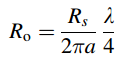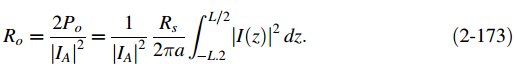### Create an Account

Already have account?

### Forgot Your Password ?

Home / Questions / Use the results of Prob 324 to calculate the radiation efficiency of a half wave dipole a...

# Use the results of Prob 324 to calculate the radiation efficiency of a half wave dipole at 100 MHz if it is made of aluminum wire 6.35 mm 025 in in diameter Assume the radiation resistance to

Use the results of Prob. 3.2-4 to calculate the radiation efficiency of a half-wave dipole at 100 MHz if it is made of aluminum wire 6.35 mm (0.25 in.) in diameter. Assume the radiation resistance to be 70 Ω.

Problem 3.2-4

Show that the ohmic resistance of a half-wave dipole from (2-173) is given byJun 22 2020 View more View Less

#### Answer (Solved)Subscribe To Get Solution